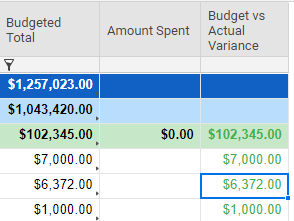# Do Not Apply Formula IF Field Is Blank

I am trying to calculate a variance by subtracting two columns but only if one of them is not blank.  I'm having trouble getting the ISBLANK condition to work.

My current formula is  =[Budgeted Total]4 - [Amount Spent]4 but I only want to apply that formula if Amount Spent is not blank.

Any suggestions would be very much appreciated!Tags:

• Try this:

=IF(ISBLANK([Amount Spent]@row), "", [Budgeted Total]@row - [Budget vs Actual Variance]@row)

• Here is a variation of my above formula in case your check column has a zero dollar placeholder:

=IF(OR(ISBLANK([Amount Spent]@row), [Amount Spent]@row = 0), "", [Budgeted Total]@row - [Budget vs Actual Variance]@row)

## Help Article Resources

Want to practice working with formulas directly in Smartsheet?

Check out the Formula Handbook template!